# Sequences & Series Questions and Answers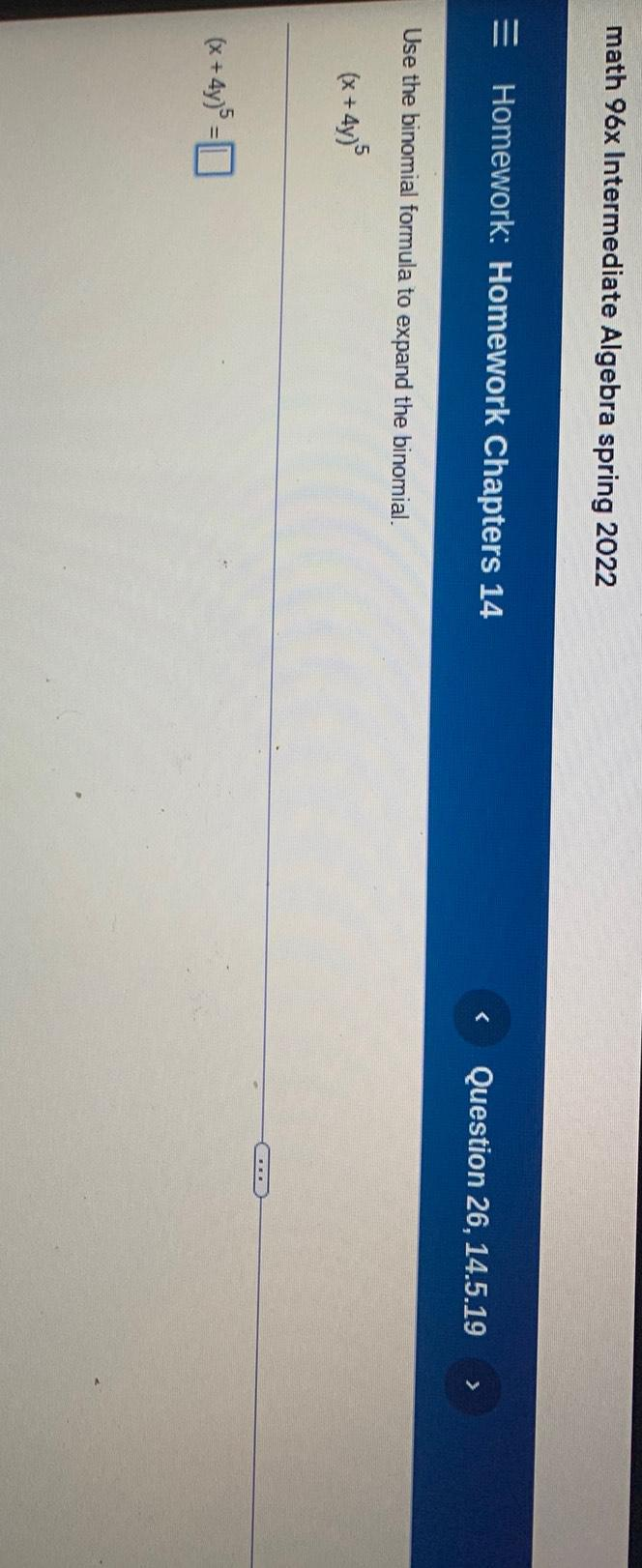Algebra
Sequences & Series
Use the binomial formula to expand the binomial. (x + 4y)⁵ (x+4y)⁵ =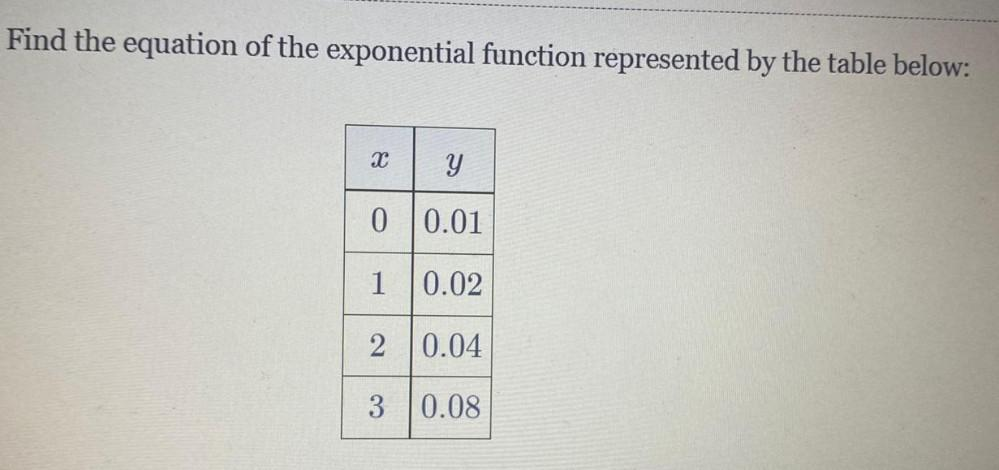Algebra
Sequences & Series
Find the equation of the exponential function represented by the table below: X Y 0 0.01 1 0.02 2 0.04 3 0.08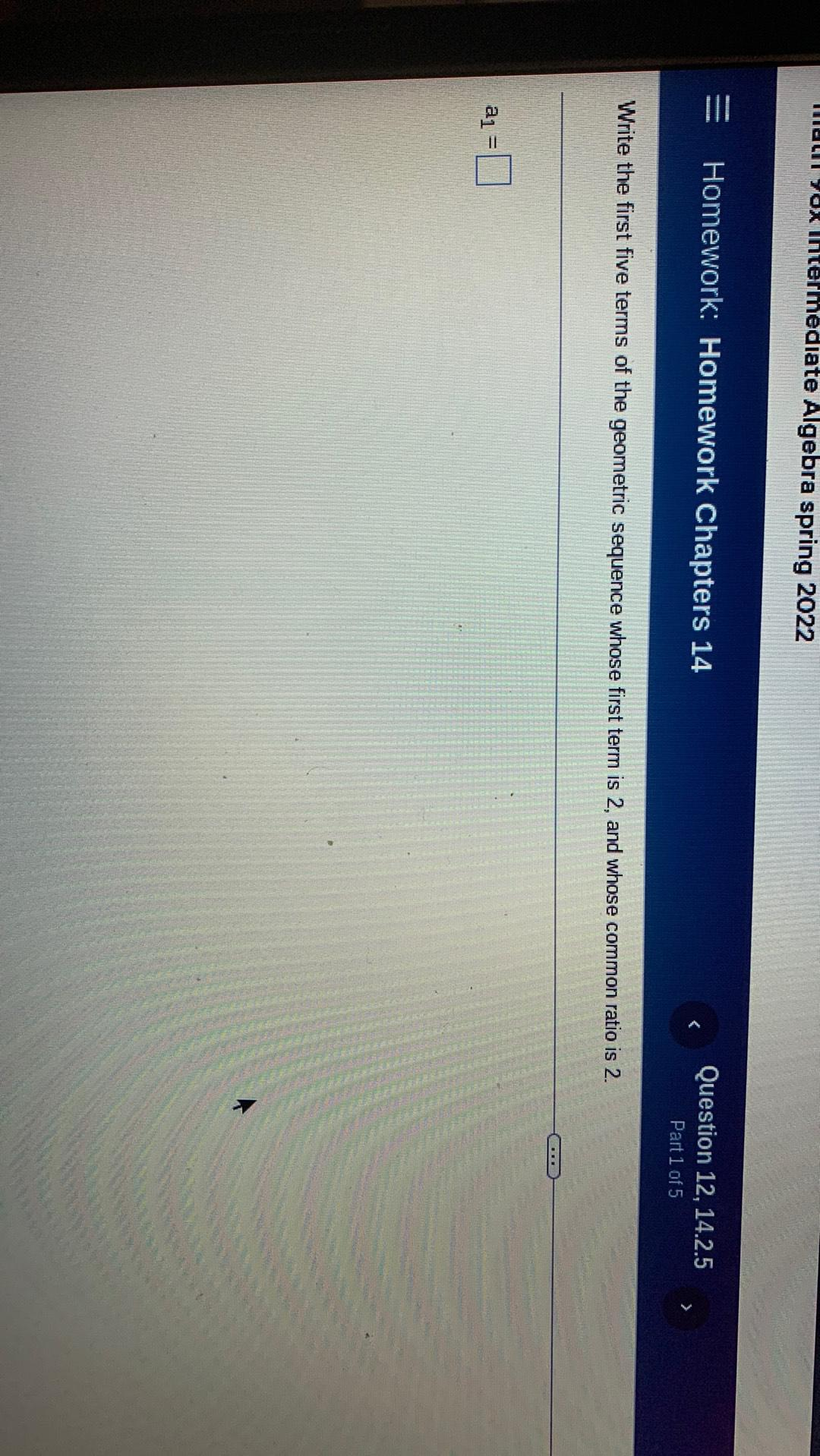Algebra
Sequences & Series
Write the first five terms of the geometric sequence whose first term is 2, and whose common ratio is 2. a1 =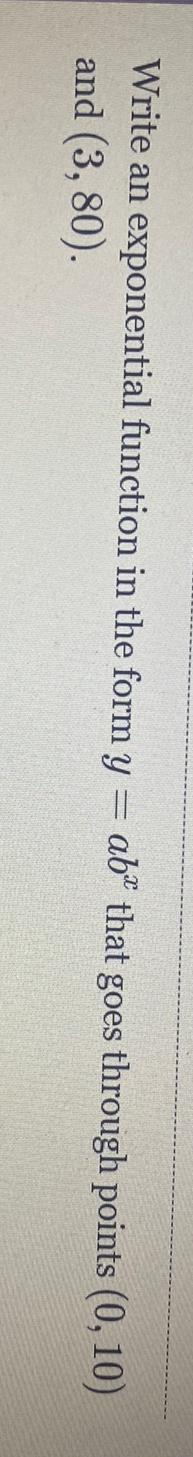Algebra
Sequences & Series
Write an exponential function in the form y = ab^x that goes through points (0, 10) and (3, 80).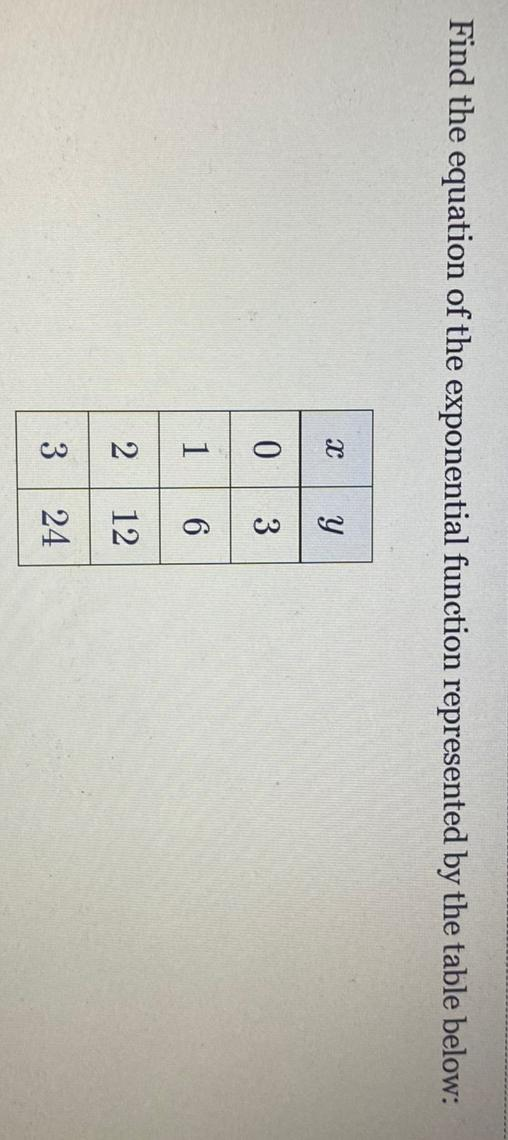Algebra
Sequences & Series
Find the equation of the exponential function represented by the table below: X Y 0 3 1 6 2 12 3 24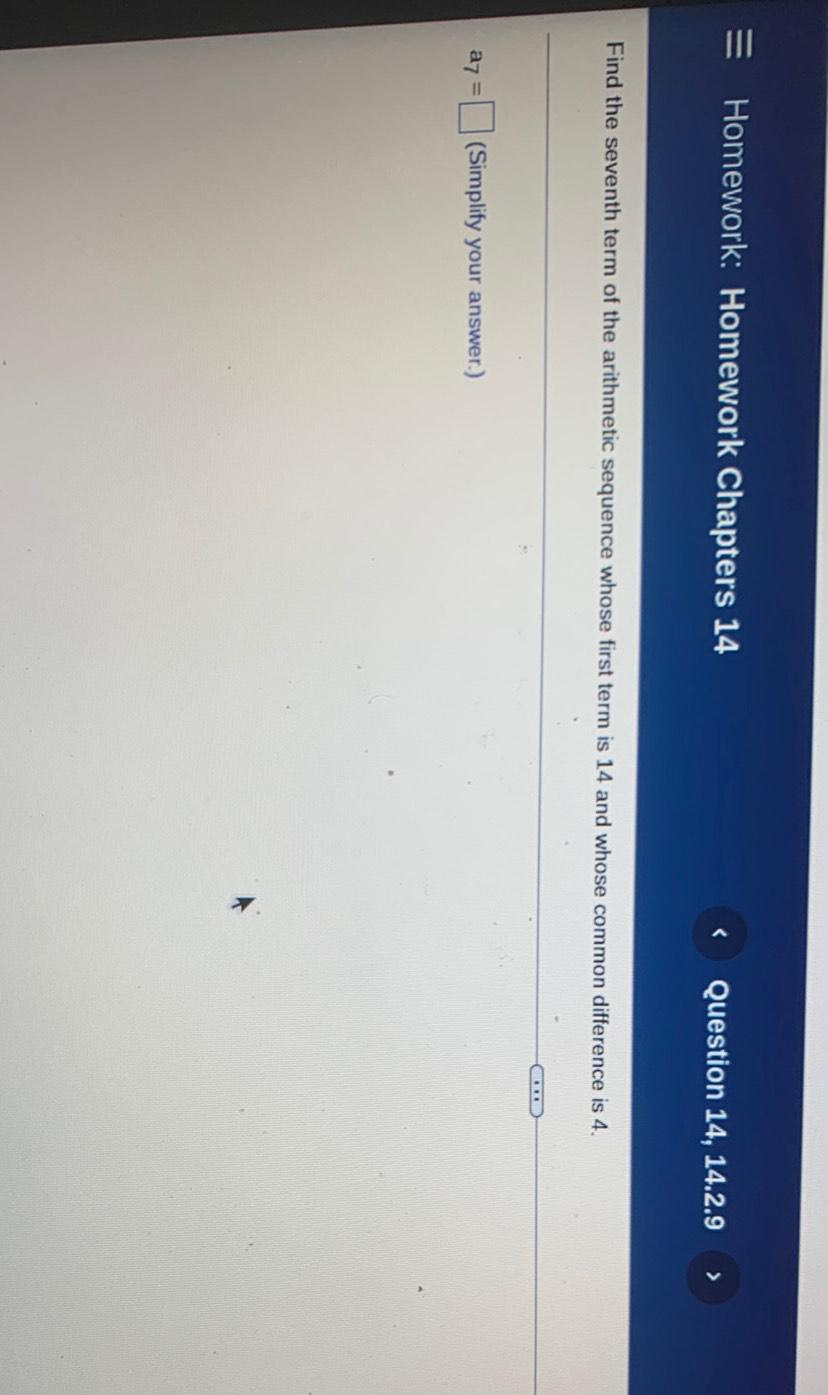Algebra
Sequences & Series
Find the seventh term of the arithmetic sequence whose first term is 14 and whose common difference is 4.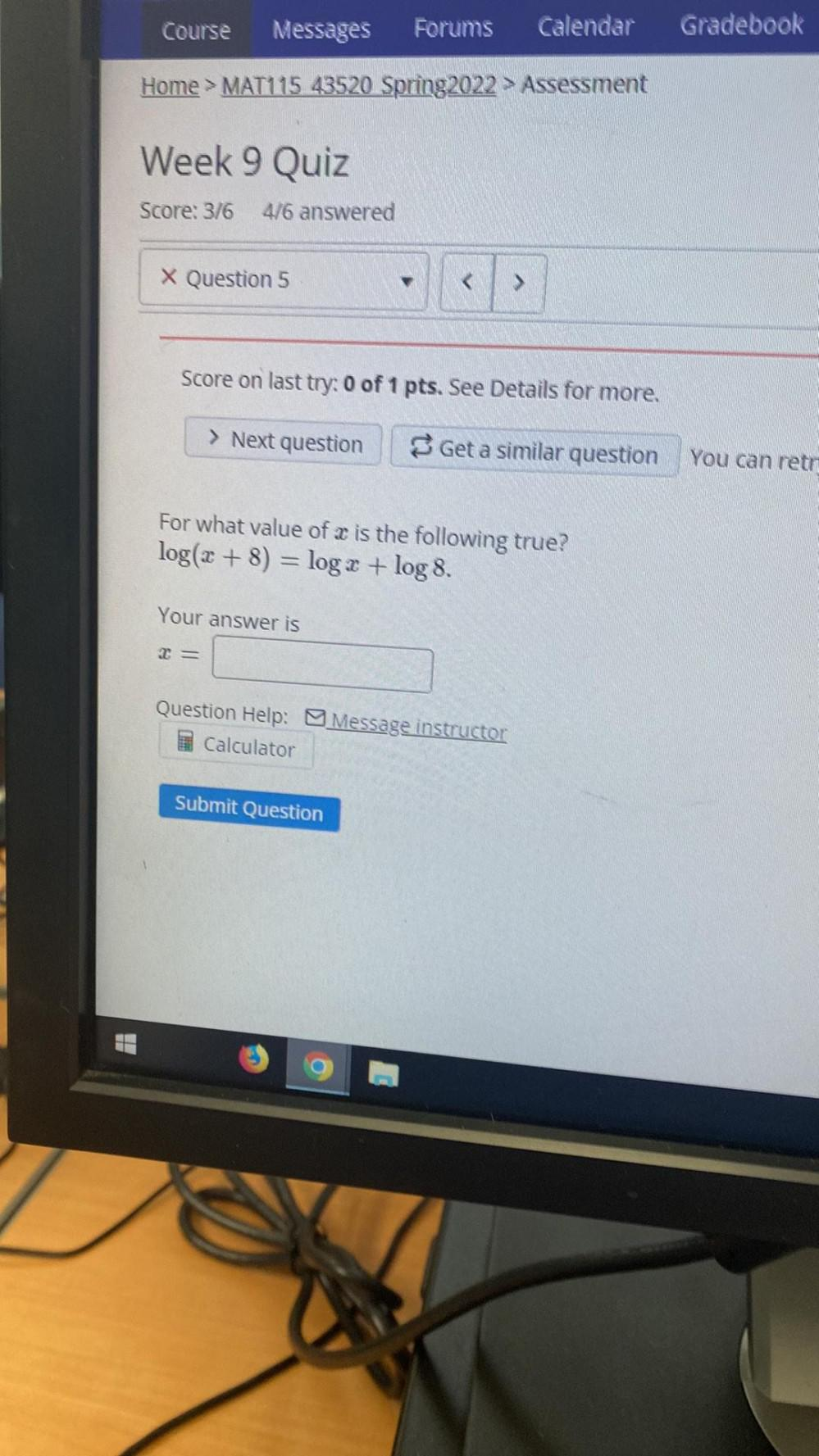Algebra
Sequences & Series
For what value of a is the following true? log(x + 8) = log x + log 8.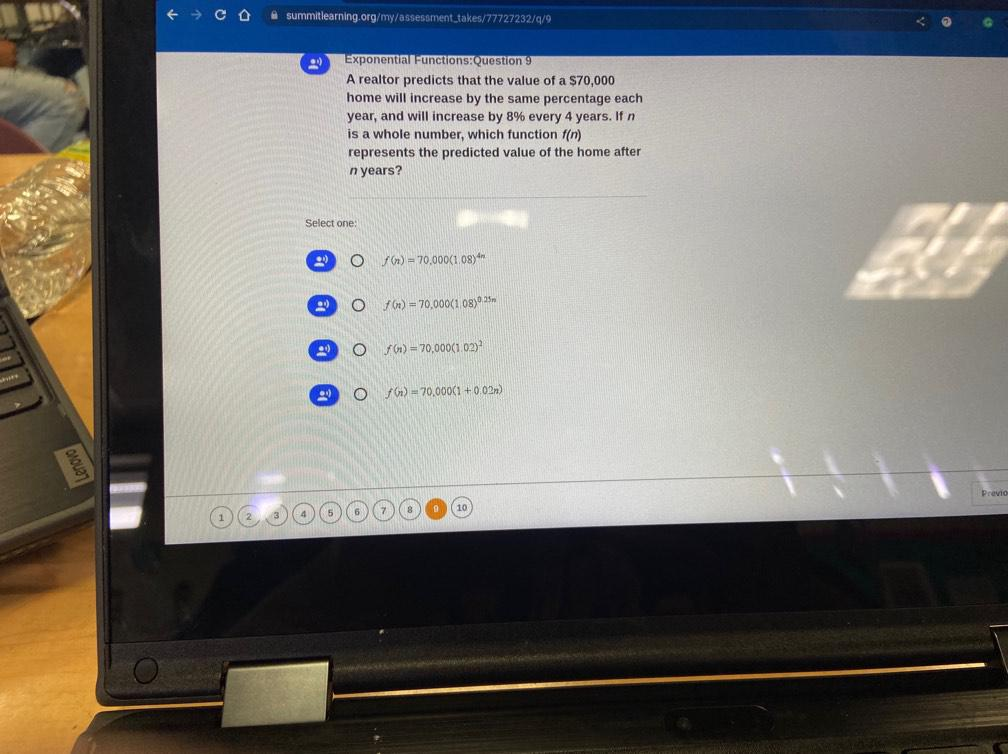Algebra
Sequences & Series
A realtor predicts that the value of a \$70,000 home will increase by the same percentage each year, and will increase by 8% every 4 years. If n is a whole number, which function f(n) represents the predicted value of the home after n years? f(n)=70,000(1.08)^(4n) f(x)=70,000(1.08)(0.25n) f(n)=70,000(1.02)^2 f(n)=70,000(1+0.02n)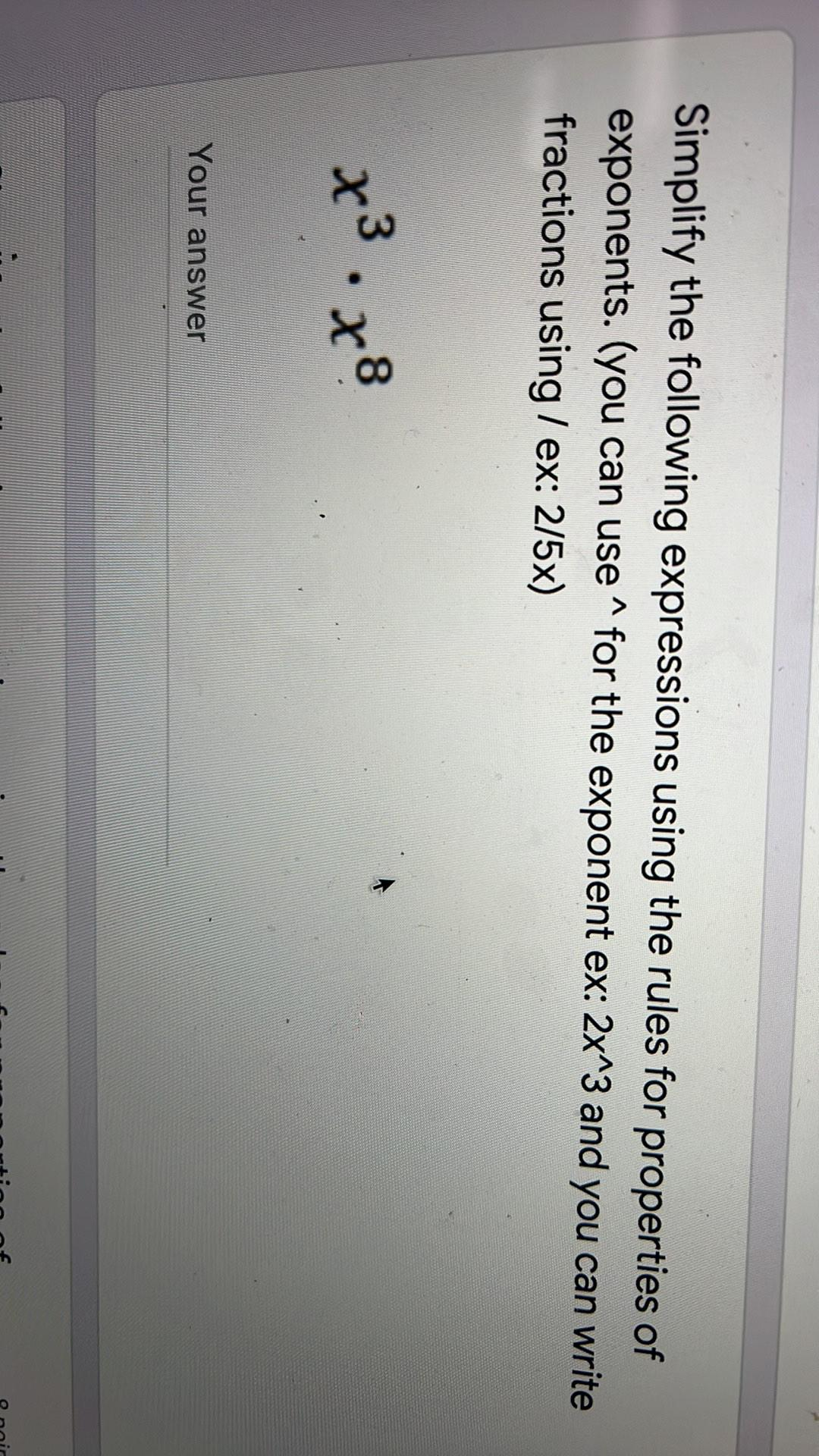Algebra
Sequences & Series
Simplify the following expressions using the rules for properties of exponents. (you can use ^ for the exponent ex: 2x^3 and you can write fractions using/ex: 2/5x) x³ . x⁸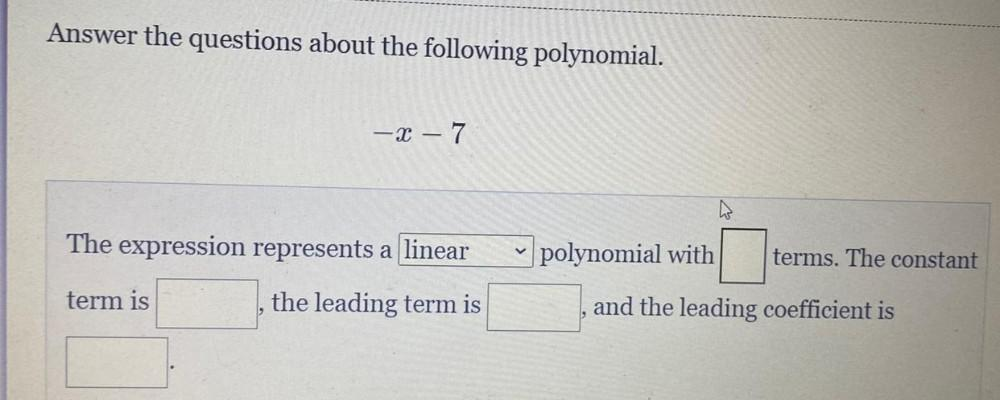Algebra
Sequences & Series
Answer the questions about the following polynomial. -x-7 The expression represents a ________ polynomial with _________ term . The constant term is _________ , the leading term is _________,and the leading coefficient is __________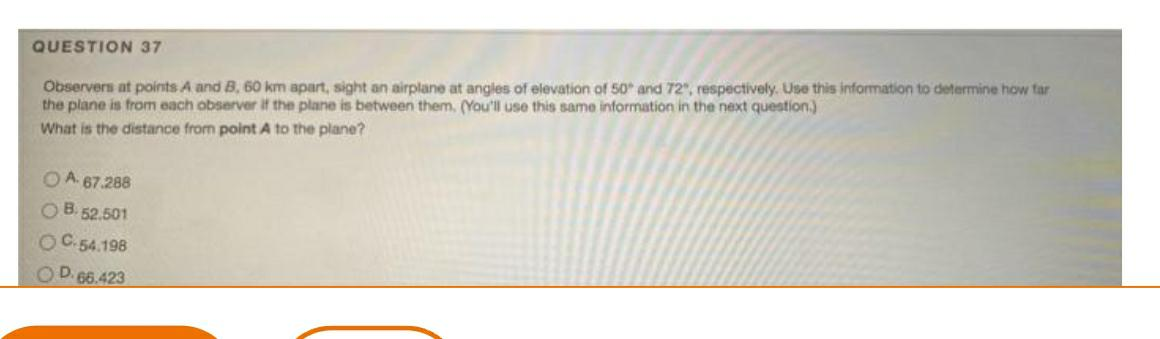Algebra
Sequences & Series
Observers at points A and B, 60 km apart, sight an airplane at angles of elevation of 50° and 72", respectively. Use this information to determine how far the plane is from each observer if the plane is between them. (You'll use this same information in the next question.) What is the distance from point A to the plane? A. 67.288 B. 52.501 C.54.198 D. 66.423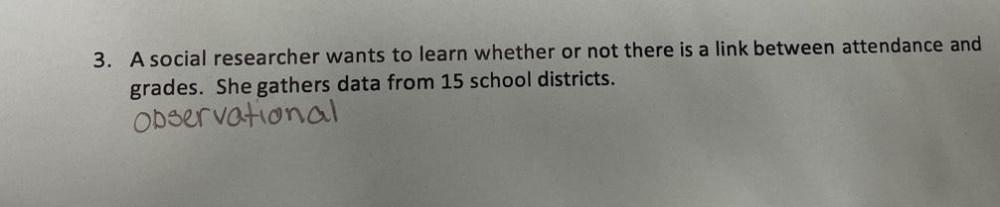Algebra
Sequences & Series
A social researcher wants to learn whether or not there is a link between attendance and grades. She gathers data from 15 school districts.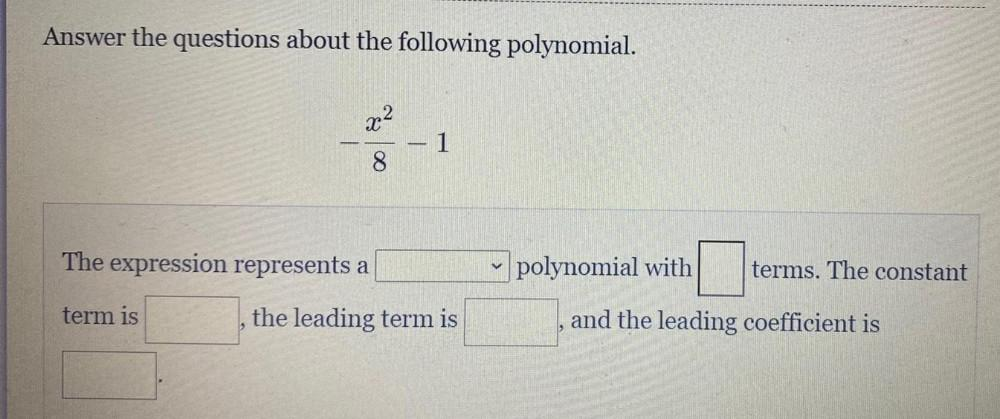Algebra
Sequences & Series
Answer the questions about the following polynomial. -(X^2/8) -1 The expression represents a_______ polynomial with______ terms. The constant term is_______ , the leading term is________ ,and the leading coefficient is ______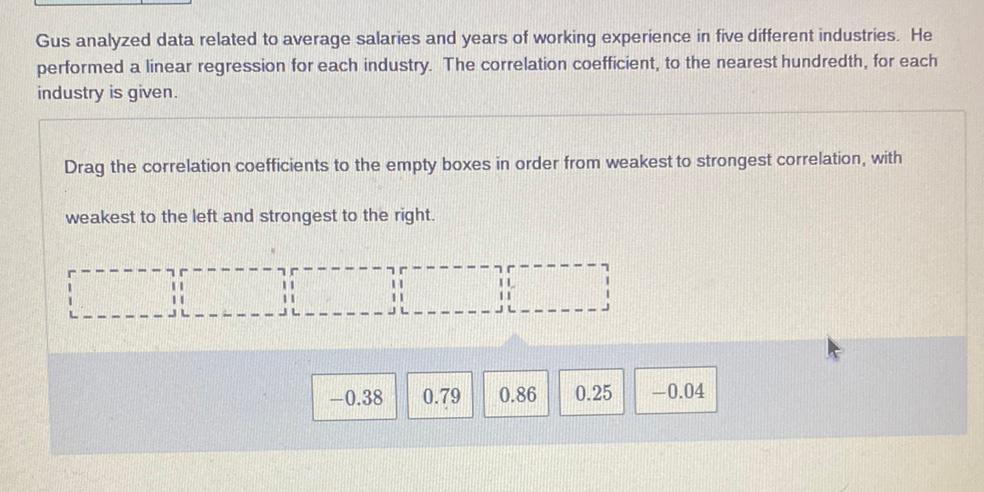Algebra
Sequences & Series
Gus analyzed data related to average salaries and years of working experience in five different industries. He performed a linear regression for each industry. The correlation coefficient, to the nearest hundredth, for each industry is given. Drag the correlation coefficients to the empty boxes in order from weakest to strongest correlation, with weakest to the left and strongest to the right.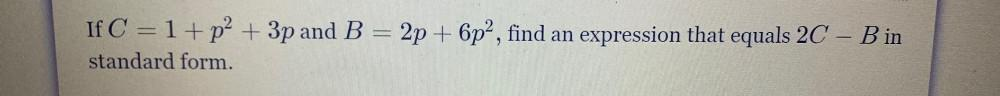Algebra
Sequences & Series
If C = 1+p² + 3p and B = 2p + 6p², find an expression that equals 2C -B in standard form.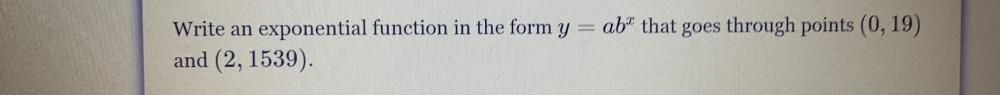Algebra
Sequences & Series
Write an exponential function in the form y=abˣ that goes through points (0,19) and (2, 1539).